KINGEXCEL.INFO ( KING OF EXCEL ) Welcome KINGEXCEL.INFO - Nothing Is Unable ... About Excel Tricks, Learning VBA Programming, Dedicated Software, Accounting, Living Skills ...

## Monday, September 21, 2020

There is an option to change the format of a range to text.
Doing this will treat any data you enter as text values, even if they are numbers. This will cause Excel to keep any leading zeros in your numbers.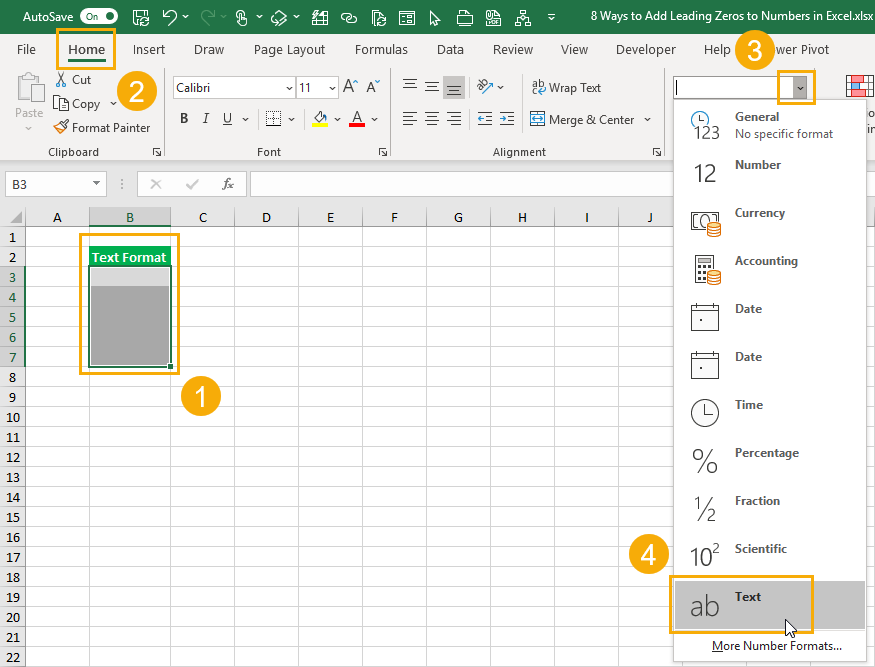Change the format from General to Text.
1. Select the range of cells you want to enter leading zeros in.
2. Go to the Home tab.
3. In the Numbers section click on the Format Dropdown selection.
4. Choose Text from the format options.
Now if you try to enter numbers with leading zeros, they won’t disappear because they are entered as text values instead of numbers.

## Custom Format

You can add a custom formatting to format numbers with leading zeros.
They will only appear to have leading zeros though. The underlying data won’t be changed into text with the added zeros.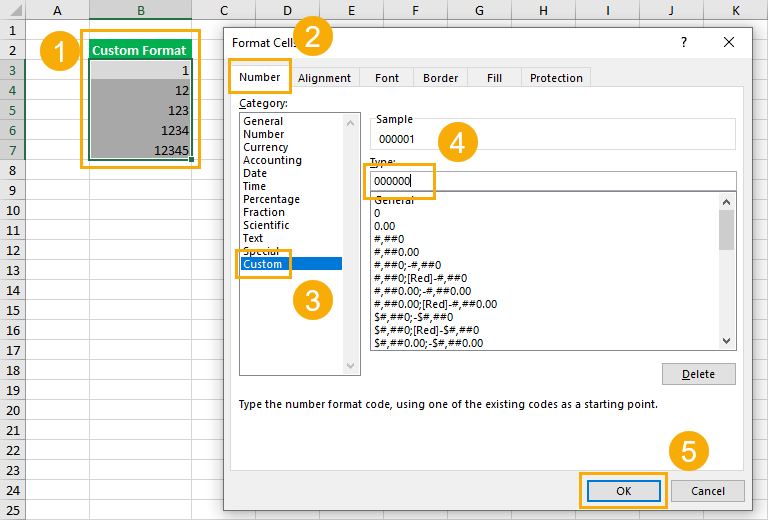1. Select the range of cells you want to add leading zeros to and open up the Format Cells dialog box.
• Right click and choose Format Cells.
• Use the Ctrl + 1 keyboard shortcut.
2. Go to the Number tab.
3. Select Custom from the category options.
4. Add a new custom format in the Type input. If you want the total number of digits including any leading zeros to be 6 then add 000000 as the custom format.
5. Press the OK button.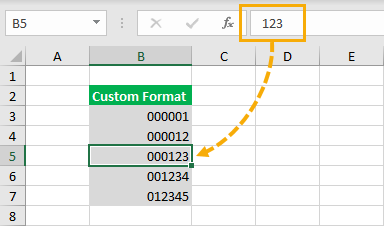After applying the custom format you will notice the data has not actually been changed.
Selecting a cell with formatting will still show the original number in the formula bar. They only appear in the worksheet with the leading zero format.
This also means if you copy and paste the data as values, you will lose the leading zeros.

You can force Excel to enter a number as text by using a leading apostrophe.
This means you’ll be able to keep those zeros in front as you’re entering your data.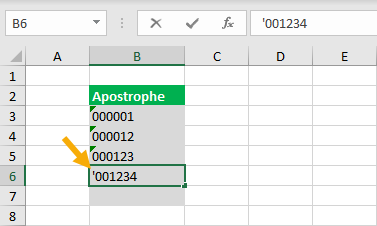This method is quick and easy while entering data. Just type a `'` character before any numbers. This will tell Excel the data is meant to be text and not a number.
When you press Enter, the leading zeros will stay visible in the worksheet. The `'` will not be visible in the worksheet, but is still there and can be seen in the formula bar when the active cell cursor is on the cell.

## TEXT Function

The TEXT function will let you apply a custom formatting to any number data already in your spreadsheet.
``= TEXT ( Value, Format)``
• Value – This is the value you want to convert to text and apply formatting to.
• Format – This is the formatting to apply.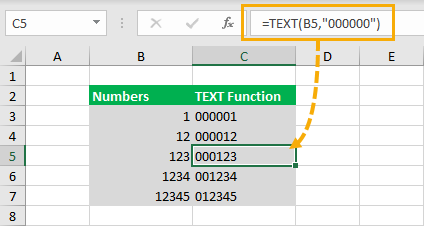``= TEXT ( B3, "000000" )``
If you wanted to add zeros to a number in cell B3 so that the total number of digits is 6, then you can use the above formula.

## RIGHT Function

Another way to get your zeros in front with a formula is using the RIGHT function. You can concatenate a string of zeros to the number and then slice off the extras using the RIGHT function.
The RIGHT function will extract the right most N characters from a text value.
``= RIGHT ( Text, [Number])``
• Text – This is the text you want to extract characters from.
• Number (Optional)- This is the number of characters to extract from the text. If this argument is not entered, then only the first character will be extracted.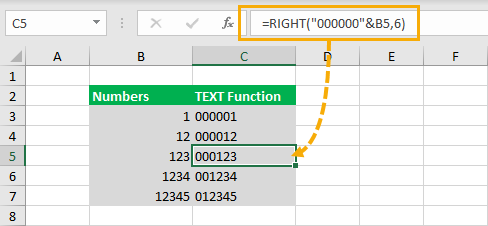``= RIGHT ( "000000" & B3, 6 )``
The above formula will concatenate several zeros to the start of a number in cell B3, then it will return the right most 6 characters resulting in some leading zeros.

## BASE Function

That’s because I didn’t know you could use the BASE function to add leading zeros until someone mentioned it in the video comments.
The BASE functions allows you to converts a number into a text representation with a given base.
The numbers you usually use are base 10, but you could use this function to convert a base 10 number into a base 2 (binary) representation.
``= BASE ( Number, Base, [MinLength])``
• Number – This is the number you want to convert to a text value in another base.
• Base – This is the base you want to convert the value to.
• MinLength (Optional) – This is the minimum length of the characters of the converted text value.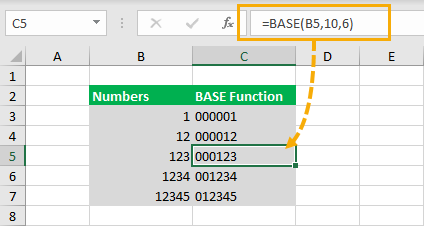``= BASE ( B3, 10, 6)``
The above formula will convert a number in cell B3 into base 10 (it’s already a base 10 number but this will keep it as base 10) and convert it to a text value with at least 6 characters. If the converted value is less than 6 characters, it will pad the value with zeros to get a minimum of 6 characters.
Definitely a cool new use for a function I otherwise never use.

## Power Query And Text.PadStart Function

Power query is all about data transformation, so it’s no surprise you can transform your numbers to add leading zeros in power query.
Unfortunately, it’s not a command that’s in the ribbon and it will require using a power query function in a custom column.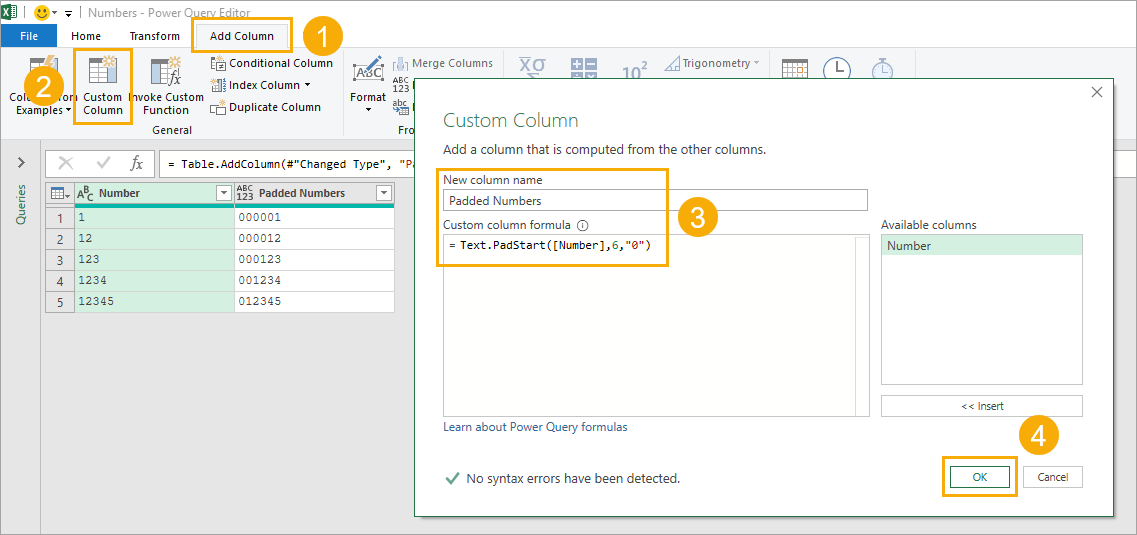``= Text.PadStart([Number],6,"0")``
After importing your data into power query, you can add a new column with the above formula to create leading zeros.
1. Go to the Add Column tab.
2. Select Custom Column from the ribbon.
3. Create a new name for the custom column and add the above formula in the Custom column formula box.
4. Press the OK button.
This will create a new column where each row has at least 6 characters padded by zeros if needed.

## Pivot Table And DAX Measure

If you want to add leading zeros to the numbers in your pivot table, you can do so by using the data model and some DAX measures.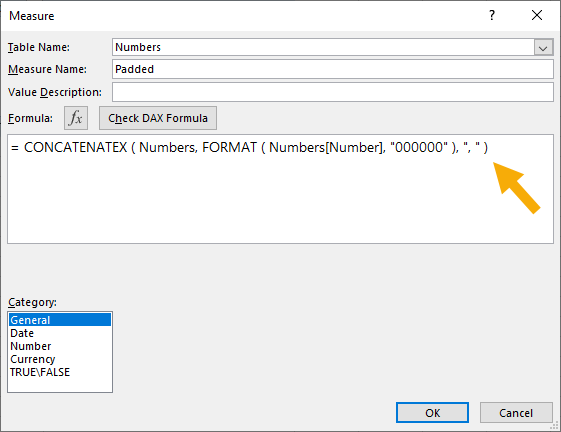``= CONCATENATEX ( Numbers, FORMAT ( Numbers[Number], "000000" ), ", " )``
You can add the above measure formula to display your numbers in a pivot table with leading zeros.
Notice the FORMAT DAX function is exactly like Excel’s TEXT function, but DAX measures need to aggregate to a single value so you need to wrap it inside a CONCATENATEX function.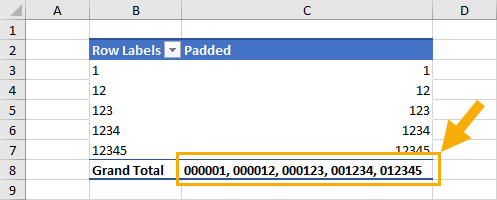When you add the new measure into the Values area of a pivot table it will result in a comma separated list of all the numbers with the leading zeros added.

## Power Pivot Calculated Column

There is another option to add zeros into you pivot table. You can add them into a calculated column in Power Pivot.
This way you can use the new column in the FilterRows or Column area of a pivot table.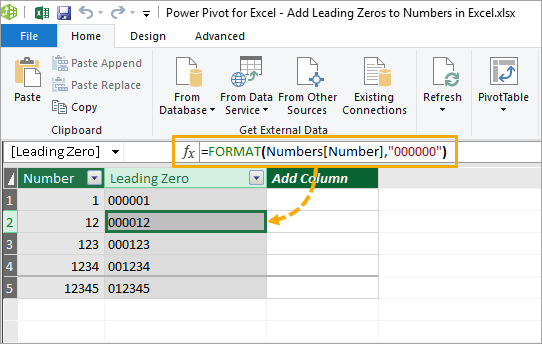``= FORMAT ( Numbers[Number], "000000" )``
In the Power Pivot add-in, you can add a new column and use the FORMAT function to create leading zeros in the column with a formula like above.
A calculated column calculates a value for each row, so there is no need to wrap the function inside a CONCATENATEX function like in the DAX measure.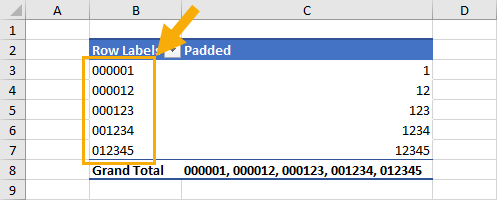Now you can use this new column inside a pivot table’s Rows area just like any other column of data!

## Conclusions

It can be frustrating to see all your zeros disappear when you don’t know why it’s happening or how to prevent it.
As you can see, there are lots of ways to make sure all those zeros stick around in your data.
Whatever your requirement, there is surely a good solution for you.
Do you have a favourite way that I missed?
#evba #etipfree #eama #kingexcel